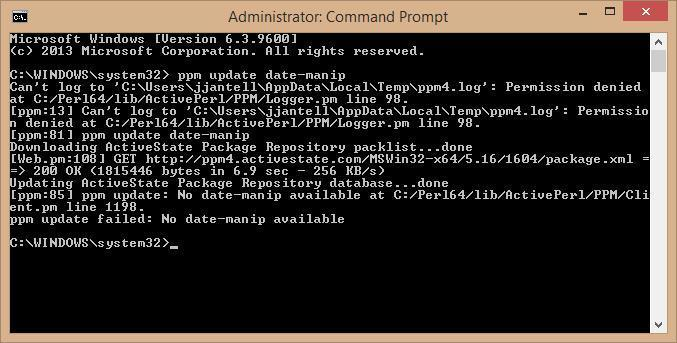If you have a series of files with names starting which have dates in this format 2014-2-6 I.E. without leading zeroes

Is it possible to do a bulk rename to add leading zeroes - e.g. 2014-02-06

You can probably write a script that can pull out the date part and reformat it in this fashion.

But if you want to use the Dynamic Renamer rename script, I just added a capability which helps to achieve this easily, so your question's timing is good.

See: Dynamic Renamer

Something like this maybe?

I thought about providing a regex, but you have this problem with simple solutions:

2014-11-2

[quote="MrC"]I thought about providing a regex, but you have this problem with simple solutions:

2014-11-2[/quote]
Ah, well spotted! I did wonder why you didn't post a RegExp solution. Is there a "one or more character" option using \d in RE?

There is. You can use quantifiers:

{1,2}

But then you run into the problem of when to add the leading 0.

Date formatting unfortunately is non-trivial with REs.

[quote="MrC"]There is. You can use quantifiers:

{1,2}

But then you run into the problem of when to add the leading 0.

Date formatting unfortunately is non-trivial.[/quote]
Thanks, that's useful. I see the problem now, I'll leave it for the scripting guys.

@jjantell If you haven't tried it yet, MrC's Dynamic Renamer is superb and well worth trying. It will solve this problem and many more.

[quote="MrC"]You can probably write a script that can pull out the date part and reformat it in this fashion.

But if you want to use the Dynamic Renamer rename script, I just added a capability which helps to achieve this easily, so your question's timing is good.

This looks just what I am after. However I am having problems installing the script (bear in mind that I have not installed a rename script before so some I may be doing something fairly basic wrong).

I have installed Pearl apparently OK. Then I come to the part of your instructions which refer to a module update and this is what I get:You can do it with just a regexp:

Old Name: ^(\d\d\d\d)(-\d\d)?-(\d)([^\d].*)\$#
New Name: \1\2-0\3\4

Thanks Leo. This works perfectly.

I have looked at the REGEX and I get the gist of how it might work but it is too complex for me to follow fully.

How ever did you work it out?

Leo is either a genius or an idiot savantJust noticed I could simplify it slightly by joining the last two groups:

Old Name: ^(\d\d\d\d)(-\d\d)?-(\d[^\d].*)\$#
New Name: \1\2-0\3

It's easier to understand if you think of it as two regexps applied one after the other.

Removing the ^ and \$ (which just ensures it matches the full filename; the \$ probably isn't strictly needed) to simplify things further, and the # from the end (more on that in a second):

[ul][li]First:

Old Name: (\d\d\d\d)-(\d[^\d].)
New Name: \1-0\2

That'll turn "2014-6x" into "2014-06x" (where x is anything that doesn't start with another digit).

[/li]
[li]Then:

Old Name: (\d\d\d\d)(-\d\d)-(\d[^\d].
)
New Name: \1\2-0\3

That'll turn "2014-06-2x" into "2014-06-02x" (where x is anything that doesn't start with another digit).[/li][/ul]

The difference between them is the extra (-\d\d) that skips the month number, if it's already two digits.

If you make the (-\d\d) optional by putting a ? after it then you have a regexp that will correct the first single-digit number after the year, whether it's in the months or days position:

Old Name: (\d\d\d\d)(-\d\d)?-(\d[^\d].*)
New Name: \1\2-0\3

That works if only one of the numbers is missing the zero. To make it add the zeros when they are missing from both numbers, all that's left is to put # on the end, to tell Opus to repeat the regexp until it stops having an effect:

Old Name: (\d\d\d\d)(-\d\d)?-(\d[^\d].*)#
New Name: \1\2-0\3

Marvellous!

Is there any way in a REGEX of converting month number to alphabetic - e.g. 2014-02-28 to 2014-FEB-08

Scripts are better for that kind of thing:

[code]@script vbscript
Option Explicit

Dim re
Set re = New RegExp
re.IgnoreCase = True
re.Pattern = "^(\d\d\d\d)-(\d\d?)-(\d\d?)([^\d]*)\$"

Function OnGetNewName ( ByRef GetNewNameData )
Dim matches, d, m, y, r
Set matches = re.Execute(GetNewNameData.item.name)
If (matches.Count > 0) Then
y = matches(0).SubMatches.Item(0)
m = matches(0).SubMatches.Item(1)
d = matches(0).SubMatches.Item(2)
r = matches(0).SubMatches.Item(3)
m = UCase(MonthName(m,True))
If (Len(d) = 1) Then d = "0" & d
OnGetNewName = y & "-" & m & "-" & d & r
End If
End Function[/code]

Which version of Opus?

10

This version may work in Opus 10, although I have only checked in 11:

[code]@script vbscript
Option Explicit

Dim re
Set re = New RegExp
re.IgnoreCase = True
re.Pattern = "^(\d\d\d\d)-(\d\d?)-(\d\d?)([^\d]*)\$"

Function Rename_GetNewName ( strFileName, strFullPath, fIsFolder, strOldName, ByRef strNewName )
Dim matches, d, m, y, r
Set matches = re.Execute(strNewName)
If (matches.Count > 0) Then
y = matches(0).SubMatches.Item(0)
m = matches(0).SubMatches.Item(1)
d = matches(0).SubMatches.Item(2)
r = matches(0).SubMatches.Item(3)
m = UCase(MonthName(m,True))
If (Len(d) = 1) Then d = "0" & d
strNewName = y & "-" & m & "-" & d & r
End If
End Function[/code]

[quote="leo"]You can do it with just a regexp:
Old Name: ^(\d\d\d\d)(-\d\d)?-(\d)([^\d].*)\$#
New Name: \1\2-0\3\4
[/quote]
That's awesome Leo, thanks. Is there any reason you use (\d\d\d\d) instead of (\d{4}) though?